# Question line, the input impedances for a open circuit load, a d, and an actual load...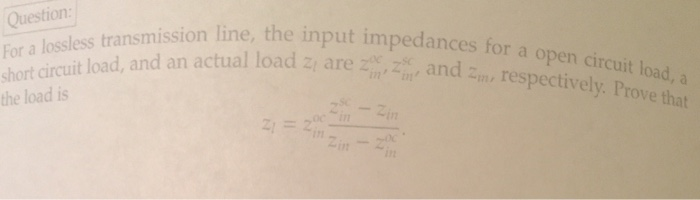Question line, the input impedances for a open circuit load, a d, and an actual load 2 are and , respectively Prove that the load is IS 2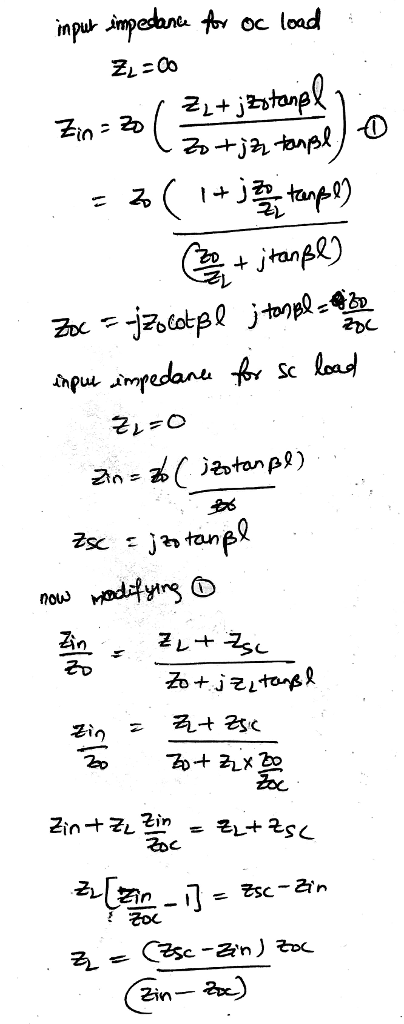#### Earn Coin

Coins can be redeemed for fabulous gifts.

Similar Homework Help Questions
• ### a. The value of the voltage source and all the load impedances of AC circuit in...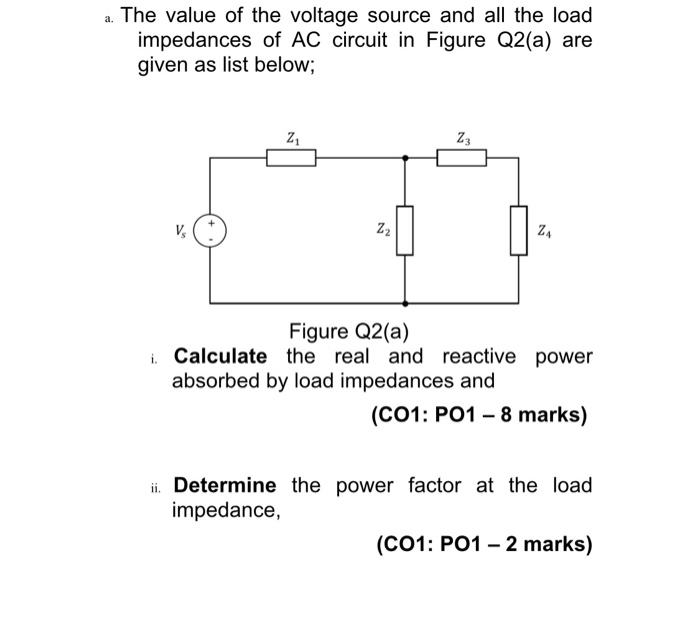a. The value of the voltage source and all the load impedances of AC circuit in Figure Q2(a) are given as list below; 21 Z3 V. Z2 ZA Figure Q2(a) Calculate the real and reactive power absorbed by load impedances and (C01: P01 - 8 marks) ii. Determine the power factor at the load impedance, (C01: P01 - 2 marks) a. The value of the voltage source and all the load impedances of AC circuit in Figure Q2(a) are given...

• ### 2. Find the reflection coefficient, SWR, and the input impedance at the distance & from the...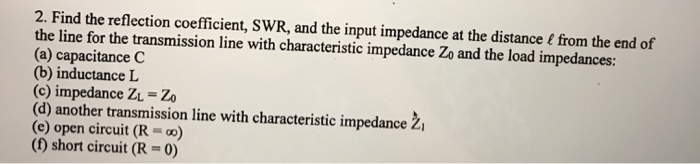2. Find the reflection coefficient, SWR, and the input impedance at the distance & from the end of the line for the transmission line with characteristic impedance Zo and the load impedances: (a) capacitance C (b) inductance L (c) impedance ZĻ = Zo (d) another transmission line with characteristic impedance Ž (e) open circuit (R = ) (f) short circuit (R = 0)

• ### ELE2601/101/3/2019 QUESTION 4 A 400 V three-phase, 50 Hz system, ABC sequence supplies the following loads: A star-connected load with the following phase impedances: A delta-connected load of eq...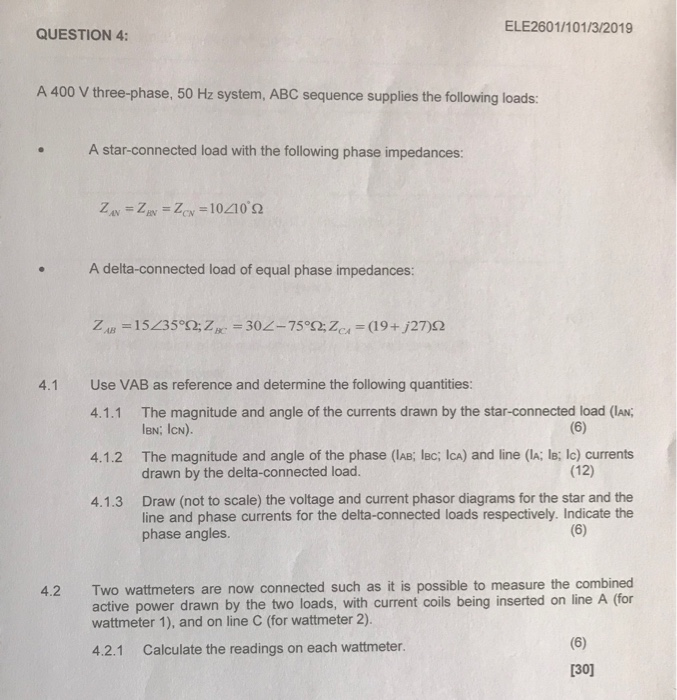ELE2601/101/3/2019 QUESTION 4 A 400 V three-phase, 50 Hz system, ABC sequence supplies the following loads: A star-connected load with the following phase impedances: A delta-connected load of equal phase impedances: Use VAB as reference and determine the following quantities: 4.1 The magnitude and angle of the currents drawn by the star-connected load (IAN; lBN; ICN). 4.1.1 The magnitude and angle of the phase (IAB; lBc; ICa) and line (lA; ls; Ic) currents drawn by the delta-connected load. 4.1.2 (12)...

• ### connected 3-phase load consisting of three identical impedances Z= 48ے36.870 W. 3-Phase System Using MATLAB/Simulink Analysis of Y-Y connected balanced 3-phase circuit using Matlab A 2400-V...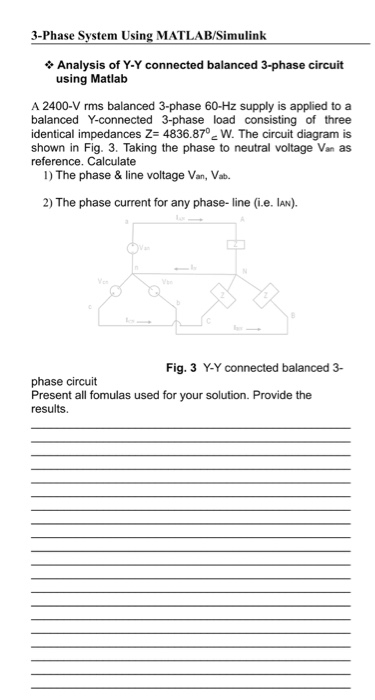connected 3-phase load consisting of three identical impedances Z= 48ے36.870 W. 3-Phase System Using MATLAB/Simulink Analysis of Y-Y connected balanced 3-phase circuit using Matlab A 2400-V rms balanced 3-phase 60-Hz supply is applied to a balanced Y-connected 3-phase load consisting of three identical impedances Z- 4836.870 W. The circuit diagram is shown in Fig. 3. Taking the phase to neutral voltage Van as reference. Calculate 1) The phase &line voltage Van, Vab 2) The phase current for any phase- line...

• ### Open/short circuit of star connected motor question help

Question: The equivalent circuit parameters of a 440 V, 3-phase, 8-poles, and 50 Hz star connected motor are given below. The short-circuit test is conducted with a locked rotor and a line current of 80 A. The open-circuit test is conducted by supplying the stator winding at rated voltage and at the same time driving the rotor in the same direction as the rotating field, at synchronous speed; s=0.R1 = 0.1, X1 = 0.5, RI2 = 0.1, XI2 = 0.5,...

• ### Question 4 (a) The input impedance of a lossless air-core transmission line with characteristic impedance Ro. phase constant B and length I terminated in an impedance Z, is given by R,+Z, tan( i....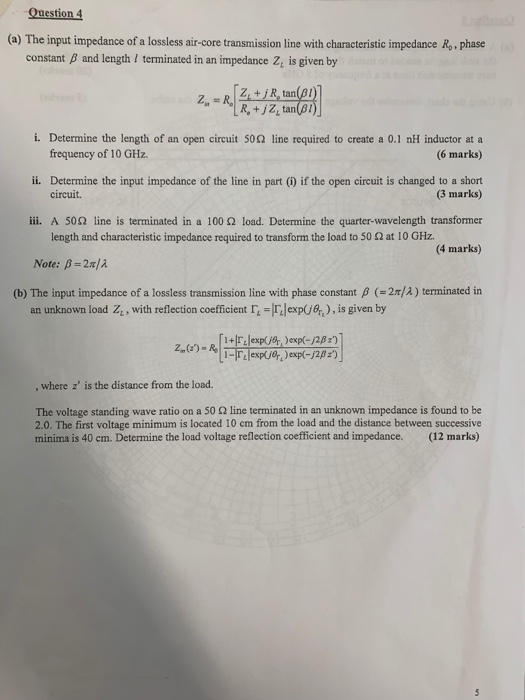Question 4 (a) The input impedance of a lossless air-core transmission line with characteristic impedance Ro. phase constant B and length I terminated in an impedance Z, is given by R,+Z, tan( i. Determine the length of an open circuit 50Ω line required to create a 0.1 nH inductor at a frequency of 10 GHz. (6 marks) ii. Determine the input impedance of the line in part () if the open circuit is changed to a short circuit. (3 marks)...

• ### 8.25 A three-phase balanced Y-connected load with series impedances of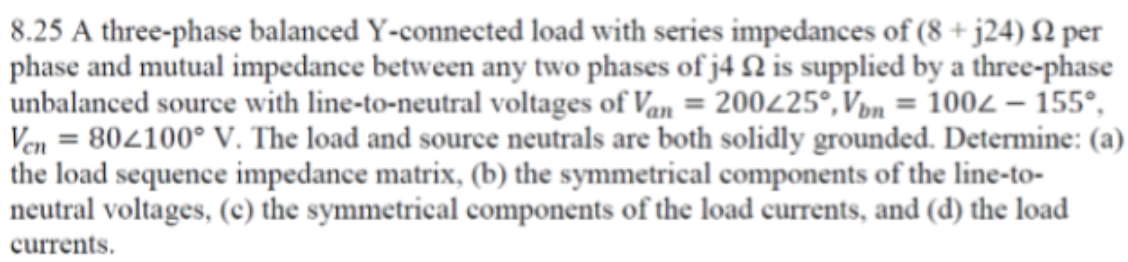8.25 A three-phase balanced $$Y$$ -connected load with series impedances of $$(8+j 24) \Omega$$ per phase and mutual impedance between any two phases of $$j 4 \Omega$$ is supplied by a three-phase unbalanced source with line-to-neutral voltages of $$V_{a n}=200 \angle 25^{\circ}, V_{b n}=100 \angle-155^{\circ}$$, $$V_{c n}=80 \angle 100^{\circ} \mathrm{V}$$. The load and source neutrals are both solidly grounded. Determine: (a) the load sequence impedance matrix, (b) the symmetrical components of the line-toneutral voltages, (c) the symmetrical components of the...

• ### What are the line currents, phase and line voltages of the load and source, respectively, for...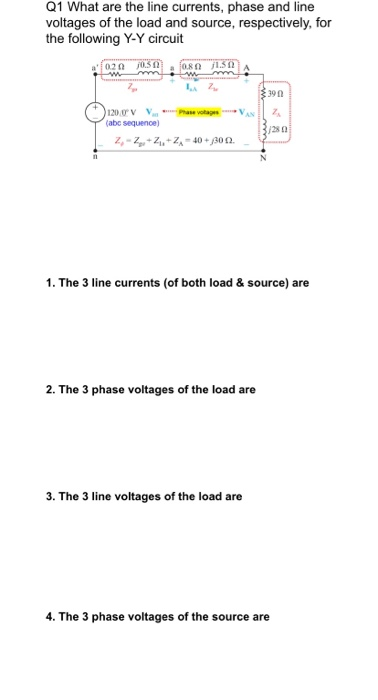What are the line currents, phase and line voltages of the load and source, respectively, for the following Y-Y circuit Q1 What are the line currents, phase and line voltages of the load and source, respectively, for the following Y-Y circuit a' 020USA a 0.8 1.SNA mm.mm 120V Vw Phase votas (abc sequence Z-Z.ZZ-40 + 300. 1. The 3 line currents (of both load & source) are 2. The 3 phase voltages of the load are 3. The 3 line...

• ### 6. Design two single-stub matching networks as shown below. Transform the load impedance Z (60 j45) Ω to match an input impedance of Z,-(75+j90)Ω. Assume that both the stub and the transmission line...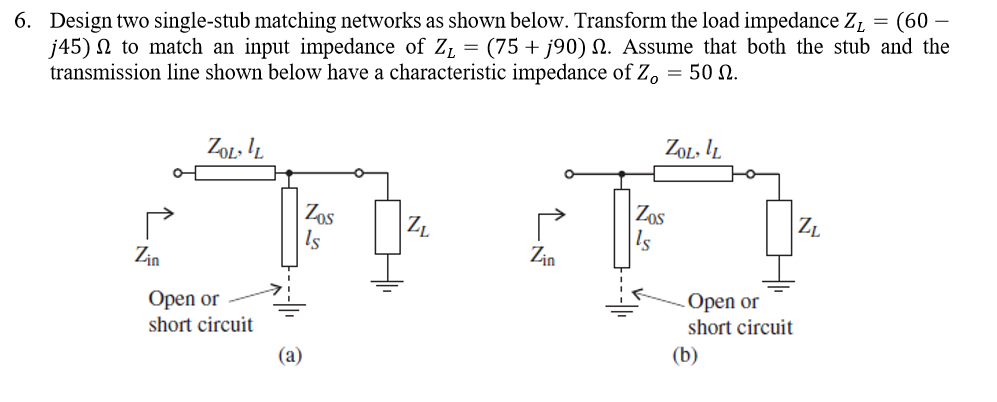6. Design two single-stub matching networks as shown below. Transform the load impedance Z (60 j45) Ω to match an input impedance of Z,-(75+j90)Ω. Assume that both the stub and the transmission line shown below have a characteristic impedance of Zo-50 Ω. Zot I ZoL.l ZL lm in Open or -) : short circuit , open or short circuit 6. Design two single-stub matching networks as shown below. Transform the load impedance Z (60 j45) Ω to match an input...

• ### Consider the transmission line circuit with given line lengths, the resistance R=500, and the line characteristic...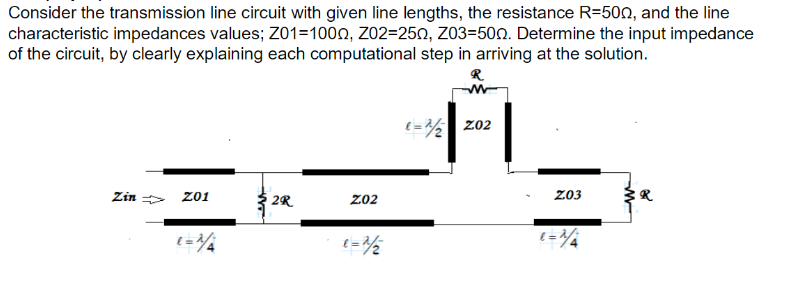Consider the transmission line circuit with given line lengths, the resistance R=500, and the line characteristic impedances values; 201=1001, 202=251, 203=508. Determine the input impedance of the circuit, by clearly explaining each computational step in arriving at the solution. R =2202 Zin 201 2R 202 203 t=1/4 t=2 =1/4